# Quiz 5: Income and Substitution Effects

a ) Let x denote 0.75-liter containers of water and y denote 2-liter containers of water. The two goods are perfect substitutes such that one unit of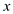gives as much utility as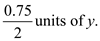Ifand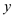are perfect substitutes such that one unit ofgives as much utility as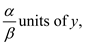then the utility function can be written as: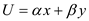Therefore, the utility function for the given scenario can be written as: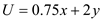b ) From the utility function, calculate the marginal utilities of the two goods.This is the case of perfect substitutes. Hence, the consumer will spend entire income onif the price ratio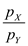is less than or equal to the ratio of marginal utilities; otherwise, zero. That is, the consumer will buy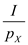units ofif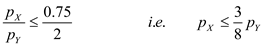Therefore, the demand function forcan be written as:c) The demand curve for goodcan be represented as the red curve in Figure 1.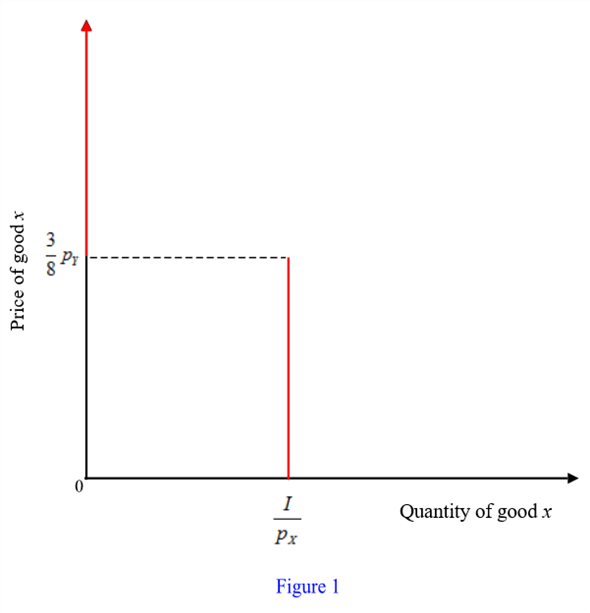d) There are two portions of the demand curve - the portion that intersects the horizontal axis and the potion that coincides the vertical axis. An increase in income will shift the former to the right. An increase in the price of goodwill increase the length of the former and reduce the length of the latter. e) The compensated demand curve shows only the substitution effect and shows no income effect. In the case of perfect substitutes, there is no income effect. Therefore, the compensated demand curve is the same as the demand curve shown in Figure 1.
Complementary goods are the goods that are consumed together by a consumer. Consumption of a single commodity do not add to the utility and thus, when the price of good increases, the demand for its complement good falls. The income of the consumer is the total budget that the individual has to spend on all the available commodities. It is given that the person D likes to consume peanut butter and jelly sandwiches using 1 ounce of jelly and 2 ounces of peanut butter. Thus, the utility and demand function of the consumer is given as perfect complements. Now it is assumed that x stands for peanut butter and y stand for jelly. The utility function for the complementary goods is as follows: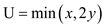The money income ( M ) of \$3 and the price of peanut butter to be \$0.05 and jelly to be \$0.10. The budget constraint is as follows: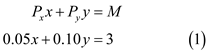Since the two goods are used in fixed proportions, therefore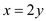. Now, it is needed to calculate the demand function. Substitute the value ofin the equation (1) as follows:Calculate the value of x as follows:Hence, person D would buy peanut butter and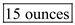of jelly. b. When the price of jelly increases to \$0.15 and it is needed to find the quantity the consumer would buy. As the price of jelly has increased to \$0.15 and thus it is costlier than before for the consumer. The new budget constraint is as follows: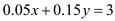Substituting the value ofto get the new consumption bundle. The new consumption bundle for the consumer will be seen as done below:Calculate the value of as follows:Due to increase in price, person D consumes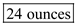of peanut butter and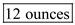of jelly. c. It is required to find the increasing amount of D's allowance in order to compensate for the rise in the price. The hike in the price of jelly has caused the consumption to fall. Thus, D must be compensated for the sacrificed units. Calculate the person D's allowance to compensation given a new income level and the change in the income level as follows:Hence, person D 's allowance to compensate would be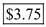. d. Represent the graph as follows: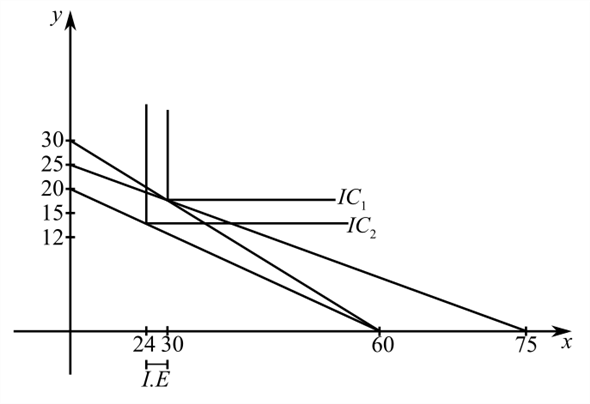All the above results are graphed in the above graph. e. It is required to graph the demand curve for the single commodity. The peanut butter and jelly are complements that are consumed together in Bread. Thus, bread consumption is the consumption of a single commodity. The units of bread consumed will stay fixed given the ounces of butter and jelly used. The demand curve shows the relationship between price and units of consumption pattern of an individual with a particular income level. Represent the graph as follows: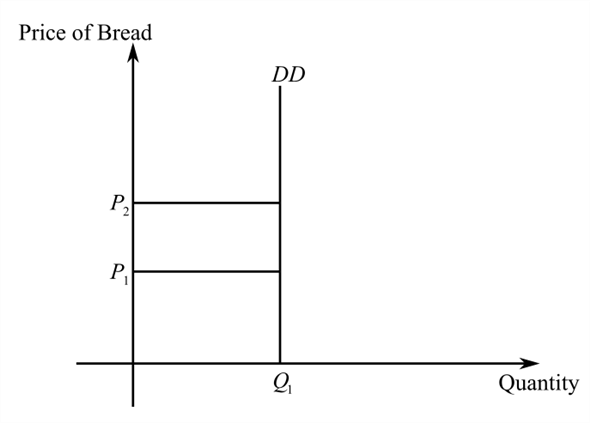The units of bread consumed will stay fixed given the ounces of butter and jelly used. The demand curve shows the relationship between price and units of consumption pattern of an individual with a particular income level. f. Substitution effect refers to the consumption of units in such a way such that the original bundle is also affordable as income is substituted to the consumer. When the price rises to \$0.15, the new income is given to be \$3.75. Calculate the new bundle purchased as follows:Calculate the value of x as follows: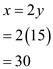Hence, the Substitution effect is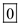. There will be no change in the quantity of goods demanded at the new income level as they are used in fixed proportions and thus, no price hike can lead to a change unless income level is not substituted in such a way that the original bundle is affordable. Income Effect refers to the change in the purchasing power of the consumer due to the change in the price level of a commodity. The consumer has the same income level, thus a hike in price level leads to a fall in purchasing power. Calculate the new consumption bundle as follows:Calculate the value of x as follows:Calculate the income effect ( I.E.) as follows:Hence, the income effect is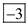.
a) It is required to be shown that if a straight line through the origin cuts all indifference curves at the points of equal slope, then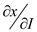is constant; that is, for any given set of prices, a given change in income always causes the same change in the quantity of goodbought/consumed. A straight line through the origin has a constant ratio of two goods at every point on the line. This means that if a straight line through the origin cuts all indifference cuts at the points of equal slope, then, MRS depends on the ratio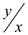of the two goods. In this case, the following expression can be written: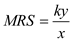where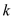is some constant For optimality, MRS should equal the price ratios. Hence,Substitute the above expression in the budget equation.Find the partial derivative of the above equation with respect to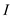.Hence, given a set of prices, the expression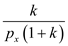is constant. Therefore,is constant. (b) Find the partial derivative of the Equation (1) with respect to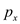.Since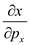is negative. An increase in price ofcauses a decrease in the quantity demanded of good. Hence, Giffen's paradox cannot occur if a straight line through the origin cuts all indifference curves at the points of equal slope. That is, Giffen's paradox cannot occur in the case of a homothetic utility function.